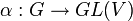# Continuous linear representation of compact group over complex numbers has invariant Hermitian inner product

## Statement

### For finite-dimensional vector space

Suppose$G$ is a Compact group (?) and$\alpha:G \to GL(V)$ (with$V$ finite-dimensional) is a Continuous linear representation (?) of$G$ over a vector space$V$ over$\mathbb{C}$, the field of complex numbers. Then, there exists a Hermitian inner product$b: V \times V \to V$ such that$\! b(\alpha(g)v,\alpha(g)w) = b(v,w) \ \forall \ g \in G, \ v,w \in V$

In other words, we can think of$b$ as a Hermitian inner product invariant under the action of$G$.

Further, if we choose a basis for$V$ that is an orthonormal basis for$b$, then in this basis, all the matrices for$\alpha(g), g \in G$, are unitary matrices, i.e., they live in the group$U(n,\mathbb{C})$ if$n$ is the dimension of$V$.